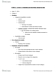# STAB22H3 Lecture Notes - Box Plot, Summary Statistics, Data Center

38 views20 pages
School
Department
Course
Professorarmindivansalar and 39413 others unlocked38
Verified Note
38 documents

## Document Summary

Stab22 lec04 (covers entire chapter 5, and some beginning part of chapter 6) Note - near the end of the lecture, he discusses data about potassium. Find: median = 17, q1= 14, q3 = 21, iqr = q3 - q1 = 21 - 14 = 7, max = 35, min = 10. Gives min, q1, median, q3 and max: Summarizes the extremes, and the percentiles (25th, 75th, 50th) => capable of summarizing large quantity of #"s w/ small quantity of summary val"s (only 5) Visual display retireved from using the following statistical summaries: Median, q3, q1, min, max, upper fence, lower fence, and iqr. => incorporates 5-number summary in a visual display. These are imaginary horizontal lines at particular values. Note - he expressed r to equal (1. 5)(iqr). So, we can alternatively write the uf, lf formulae as follows: Draw vertical lines protruding from box of boxplot to most extreme value within the.

## Get access

\$8 USD/m\$10 USD/m
Billed \$96 USD annuallyHomework Help
Study Guides
Textbook Solutions
Class Notes
Textbook Notes
Booster Class